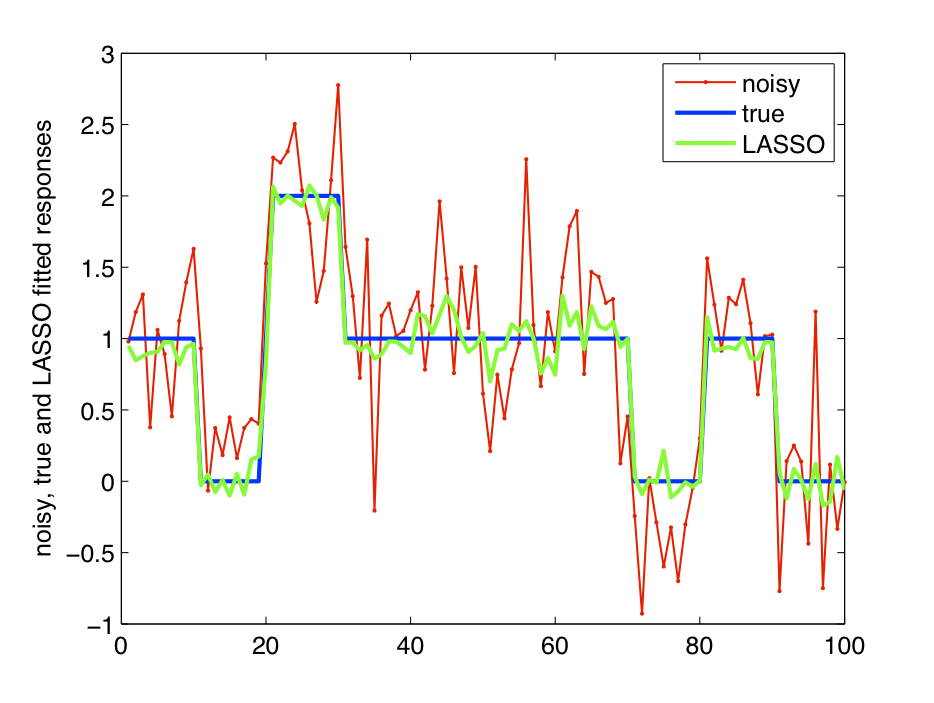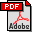## A tutorial on the LASSO and the "shooting algorithm"

### Abstract

The LASSO is an L1 penalized regression technique introduced by Tibshirani (1996). An efficient algorithm called the "shooting algorithm" was proposed by Fu (1998) for solving the LASSO problem in the multi parameter case. In this tutorial, we present a simple and self-contained derivation of the LASSO "shooting algorithm".

### Main ideas

1. LASSO is an L1 penalized linear regression procedure that regularizes the solution and results in sparsity/feature selection.

2. The LASSO objective function is convex and non-differentiable. However, there is a special structure in the non-differentiable part which makes convergence of the co-ordinate wise optimization to the global minimum possible.

3. The "shooting algorithm" is a special instance of co-ordinate wise optimization applied to the LASSO objective function.

### Key references

1. Gautam V. Pendse, "A tutorial on the LASSO and the "shooting algorithm", [pdf] (this tutorial)

2. J. Friedman, T. Hastie, H. Hofling, and R. Tibshirani. Pathwise coordinate optimization. The Annals of Applied Statistics, 1(2):302-332, 2007. [pdf]

3. W. J. Fu. Penalized Regressions: The Bridge Versus the Lasso. Journal of Computational and Graphical Statistics, 7:397-416, 1998. [pdf]

4. R. Tibshirani. Regression shrinkage and selection via the lasso. Journal of the Royal Statistical Society Series B, 58(1):267-288, 1996. [pdf]

5. P. Tseng. Coordinate Ascent for Maximizing Nondifferentiable Concave Functions. Technical Report LIDS-P-1840, Laboratory for Information and Decision Systems, Massachusetts Institute of Technology, 1988. [pdf]

6. P. Tseng. Convergence of a Block Coordinate Descent Method for Nondifferentiable Minimization. Journal of optimization theory and applications, 109(3):475-494, 2001. [pdf]

### PDF Figures

Matlab code for fitting the LASSO model and estimating the regularization parameter can be downloaded here: lasso_webpage_code_data.zip. This software is freely available under the terms of the license described below. This zip file includes the following directories:

#### webpage

This directory contains a standalone version of this webpage lasso_shooting.html for offline browsing.

#### code

This directory contains code for:
1. fitting a LASSO model: solveLasso.m

2. estimating the regularization parameter: estimateLassoLambda.m

3. reproducing Figure 1 and Figure 2 in the tutorial: exampleLassoUsage.m

#### data

This directory contains a MATLAB .mat file: sampleDataForLasso.mat which contains the data used to generate Figure 1 and Figure 2.